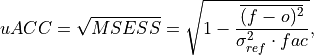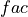# climpred.metrics._uacc#

climpred.metrics._uacc(forecast: xarray.Dataset, verif: xarray.Dataset, dim: Optional[Union[str, List[str]]] = None, **metric_kwargs: Any) [source]#

Bushuk’s unbiased Anomaly Correlation Coefficient (uACC).

This is typically used in perfect model studies. Because the perfect model Anomaly Correlation Coefficient (ACC) is strongly state dependent, a standard ACC (e.g. one computed using _pearson_r()) will be highly sensitive to the set of start dates chosen for the perfect model study. The Mean Square Skill Score (MESSS) can be related directly to the ACC as MESSS = ACC^(2) (see Murphy  and Bushuk et al. ), so the unbiased ACC can be derived as uACC = sqrt(MESSS).whereis 1 when using comparisons involving the ensemble mean (m2e, e2c, e2o) and 2 when using comparisons involving individual ensemble members (m2c, m2m, m2o). See _get_norm_factor().

Note

Because of the square root involved, any negative MSESS values are automatically converted to NaNs.

Parameters
• forecast – Forecast.

• verif – Verification data.

• dim – Dimension(s) to perform metric over.

• comparison – Name comparison needed for normalization factor fac, see _get_norm_factor() (Handled internally by the compute functions)

• metric_kwargs – see xskillscore.mse()

Notes

 minimum 0.0 maximum 1.0 perfect 1.0 orientation positive better than climatology > 0.0 equal to climatology 0.0

References

Example

>>> HindcastEnsemble.verify(
...     metric="uacc", comparison="e2o", alignment="same_verifs", dim="init"
... )
<xarray.Dataset>
Coordinates:
* lead     (lead) int32 1 2 3 4 5 6 7 8 9 10
skill    <U11 'initialized'
Data variables:
SST      (lead) float64 0.9093 0.9041 0.8849 0.877 ... 0.6894 0.5702 0.4763
Attributes: